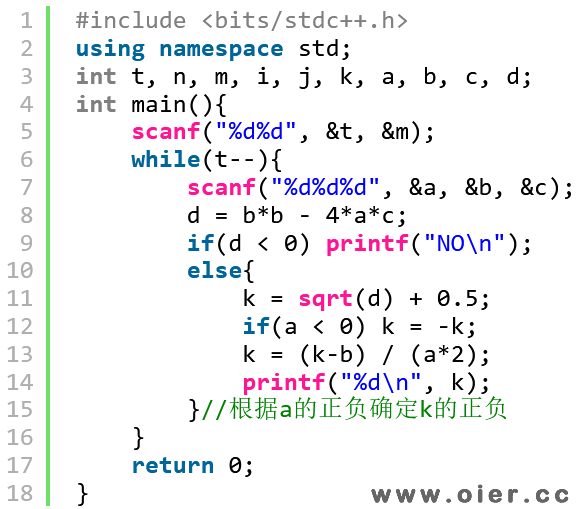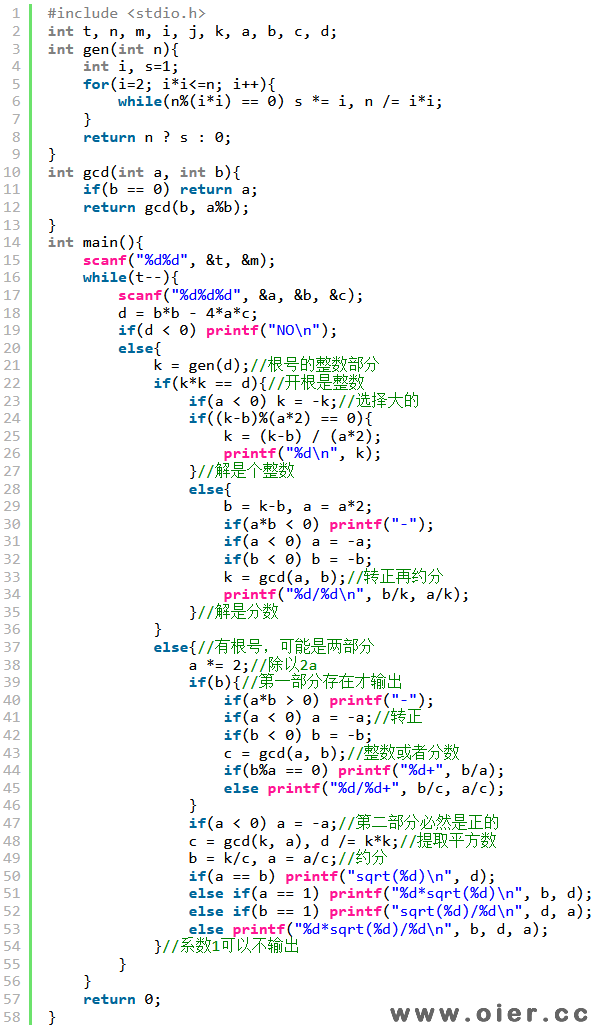158+

## 题目背景

– 计算 $\Delta = b ^ 2 – 4ac$，则:
1. 若 $\Delta < 0$，则该一元二次方程无实数解。
2. 否则 $\Delta \geq 0$，此时该一元二次方程有两个实数解 $x _ {1, 2} = \frac{-b \pm \sqrt \Delta}{2a}$。

– $x ^ 2 + x + 1 = 0$ 无实数解，因为 $\Delta = 1 ^ 2 – 4 \times 1 \times 1 = -3 < 0$。
– $x ^ 2 – 2x + 1 = 0$ 有两相等实数解 $x _ {1, 2} = 1$。
– $x ^ 2 – 3x + 2 = 0$ 有两互异实数解 $x _ 1 = 1, x _ 2 = 2$。

## 题目描述

**在本题中输出有理数 $v$ 时须遵循以下规则：**

– 由有理数的定义，存在唯一的两个整数 $p$ 和 $q$，满足 $q > 0$，$\gcd(p, q) = 1$ 且 $v = \frac pq$。
– 若 $q = 1$，**则输出 {p}，否则输出 {p}/{q}**，其中 {n} 代表整数 $n$ 的值；
– 例如：

– 当 $v = -0.5$ 时，$p$ 和 $q$ 的值分别为 $-1$ 和 $2$，则应输出 -1/2；
– 当 $v = 0$ 时，$p$ 和 $q$ 的值分别为 $0$ 和 $1$，则应输出 0。

**对于方程的求解，分两种情况讨论：**

1. 若 $\Delta = b ^ 2 – 4ac < 0$，则表明方程无实数解，此时你应当输出 NO；
2. 否则 $\Delta \geq 0$，此时方程有两解（可能相等），记其中较大者为 $x$，则：
1. 若 $x$ 为有理数，则按有理数的格式输出 $x$。
2. 否则根据上文公式，$x$ 可以被**唯一**表示为 $x = q _ 1 + q _ 2 \sqrt r$ 的形式，其中：

– $q _ 1, q _ 2$ 为有理数，且 $q _ 2 > 0$；
– $r$ 为正整数且 $r > 1$，且不存在正整数 $d > 1$ 使 $d ^ 2 \mid r$（即 $r$ 不应是 $d ^ 2$ 的倍数）；

1. 若 $q _ 1 \neq 0$，则按有理数的格式输出 $q _ 1$，并再输出一个加号 +；
2. 否则跳过这一步输出；

1. 若 $q _ 2 = 1$，则输出 sqrt({r})；
2. 否则若 $q _ 2$ 为整数，则输出 {q2}*sqrt({r})；
3. 否则若 $q _ 3 = \frac 1{q _ 2}$ 为整数，则输出 sqrt({r})/{q3}；
4. 否则可以证明存在唯一整数 $c, d$ 满足 $c, d > 1, \gcd(c, d) = 1$ 且 $q _ 2 = \frac cd$，此时输出 {c}*sqrt({r})/{d}；

## 输入输出格式

### 输出格式

**每行输出的字符串中间不应包含任何空格**。

## 输入输出样例

### 输入样例 #1

9 1000
1 -1 0
-1 -1 -1
1 -2 1
1 5 4
4 4 1
1 0 -432
1 -3 1
2 -4 1
1 7 1

### 输出样例 #1

1
NO
1
-1
-1/2
12*sqrt(3)
3/2+sqrt(5)/2
1+sqrt(2)/2
-7/2+3*sqrt(5)/2

## 说明

**【样例 #2】**

**【数据范围】**

| 测试点编号 | $M \leq$ | 特殊性质 A | 特殊性质 B | 特殊性质 C |
| :-: | :-: | :-: | :-:| :-:|
| $1$ | $1$ | 是 | 是 | 是 |
| $2$ | $20$ | 否 | 否 | 否 |
| $3$ | $10 ^ 3$ | 是 | 否 | 是 |
| $4$ | $10 ^ 3$ | 是 | 否 | 否 |
| $5$ | $10 ^ 3$ | 否 | 是 | 是 |
| $6$ | $10 ^ 3$ | 否 | 是 | 否 |
| $7, 8$ | $10 ^ 3$ | 否 | 否 | 是 |
| $9, 10$ | $10 ^ 3$ | 否 | 否 | 否 |

– 特殊性质 A：保证 $b = 0$；
– 特殊性质 B：保证 $c = 0$；
– 特殊性质 C：如果方程有解，那么方程的两个解都是整数。

## 解题思路

50分随便拿：只需要直接开根，输出整数答案即可，需要注意要根据a的正负判断正负号的取值。100分就是分类讨论模拟，最简分数用gcd约分即可，开根的话用质因数分解即可，另外就是一些细节，什么时候必须输出，什么时候不该输出。

## 程序实现